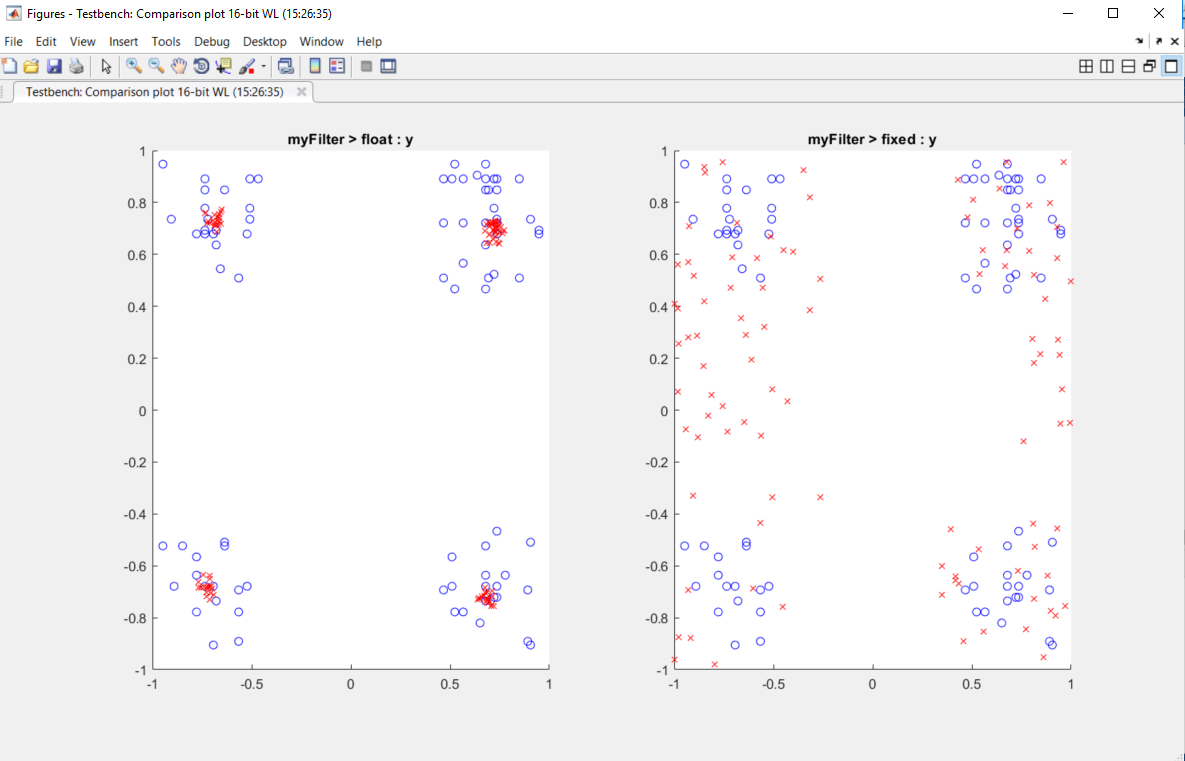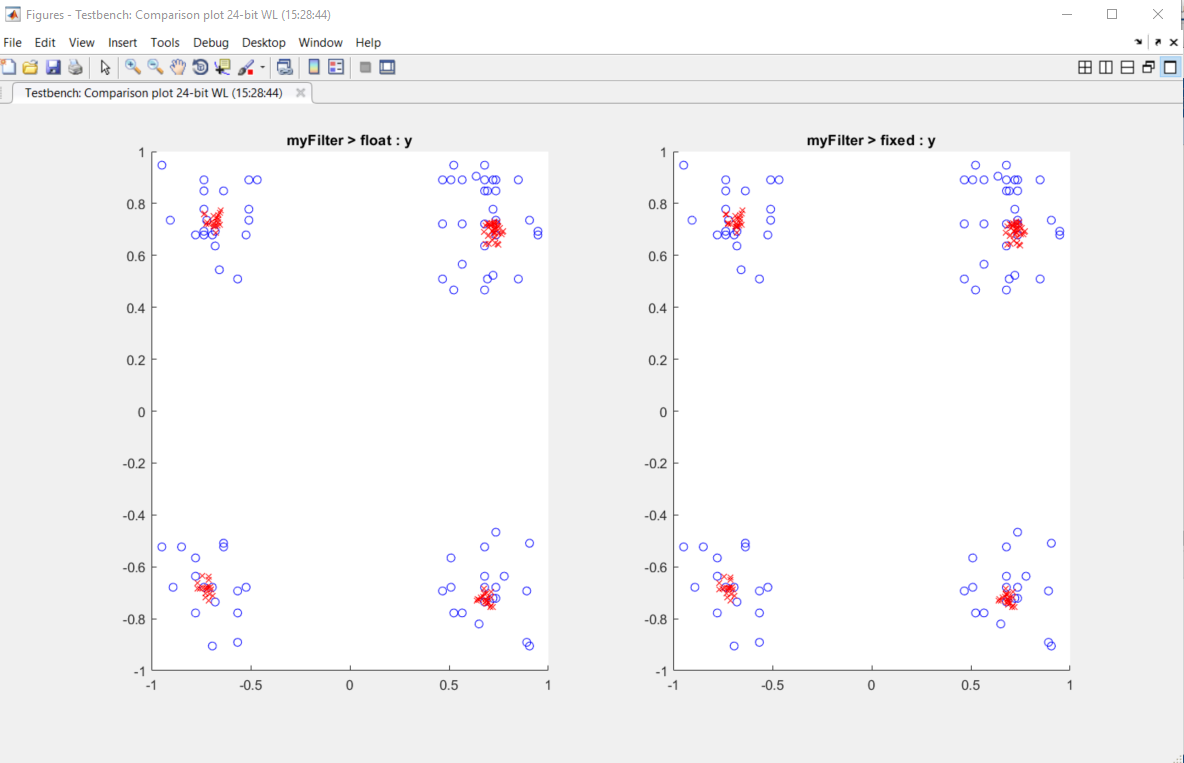Visualize Differences Between Floating-Point and Fixed-Point Results

This example shows how to configure the fiaccel function to use a custom plot function to compare the behavior of the generated fixed-point code against the behavior of the original floating-point MATLAB® code.

By default, when the LogIOForComparisonPlotting option is enabled, the conversion process uses a time series based plotting function to show the floating-point and fixed-point results and the difference between them. However, during fixed-point conversion you might want to visualize the numerical differences in a view that is more suitable for your application domain. This example shows how to customize plotting and produce scatter plots at the test numerics step of the fixed-point conversion.

Prerequisites

To complete this example, you must install the following products:

• MATLAB

• Fixed-Point Designer™

• C compiler

You can use mex -setup to change the default compiler. See Change Default Compiler.

Create a New Folder and Copy Relevant Files

1. Create a local working folder, for example, c:\custom_plot.

2. Change to the docroot\toolbox\fixpoint\examples folder. At the MATLAB command line, enter:

cd(fullfile(docroot, 'toolbox', 'fixpoint', 'examples'))

3. Copy the myFilter.m, myFilterTest.m, plotDiff.m, and filterData.mat files to your local working folder.

It is best practice to create a separate test script to do all the pre- and post-processing such as loading inputs, setting up input values, calling the function under test, and outputting test results.

TypeNameDescription
Function codemyFilter.mEntry-point MATLAB function
Test filemyFilterTest.mMATLAB script that tests myFilter.m
Plotting functionplotDiff.mCustom plot function
MAT-filefilterData.matData to filter.

Set Up Configuration Object

1. Create a coder.FixptConfig object.

fxptcfg = coder.config('fixpt');
2. Specify the test file name and custom plot function name. Enable logging and numerics testing.

fxptcfg.TestBenchName = 'myFilterTest';
fxptcfg.PlotFunction = 'plotDiff';
fxptcfg.TestNumerics = true;
fxptcfg. LogIOForComparisonPlotting = true;
fxptcfg.DefaultWordLength = 16;

Convert to Fixed Point

Convert the floating-point MATLAB function, myFilter, to fixed-point MATLAB code. You do not need to specify input types for the fiaccel command because it infers the types from the test file.

fiaccel -args {complex(0, 0)} -float2fixed fxptcfg myFilter

The conversion process generates fixed-point code using a default word length of 16 and then runs a fixed-point simulation by running the myFilterTest.m function and calling the fixed-point version of myFilter.m.

Because you selected to log inputs and outputs for comparison plots and to use the custom plotting function, plotDiff.m, for these plots, the conversion process uses this function to generate the comparison plot.The plot shows that the fixed-point results do not closely match the floating-point results.

Increase the word length to 24 and then convert to fixed point again.

fxptcfg.DefaultWordLength = 24;
fiaccel -args {complex(0, 0)} -float2fixed fxptcfg myFilter

The increased word length improved the results. This time, the plot shows that the fixed-point results match the floating-point results.Fixed-Point Designer DocumentationGet trial now